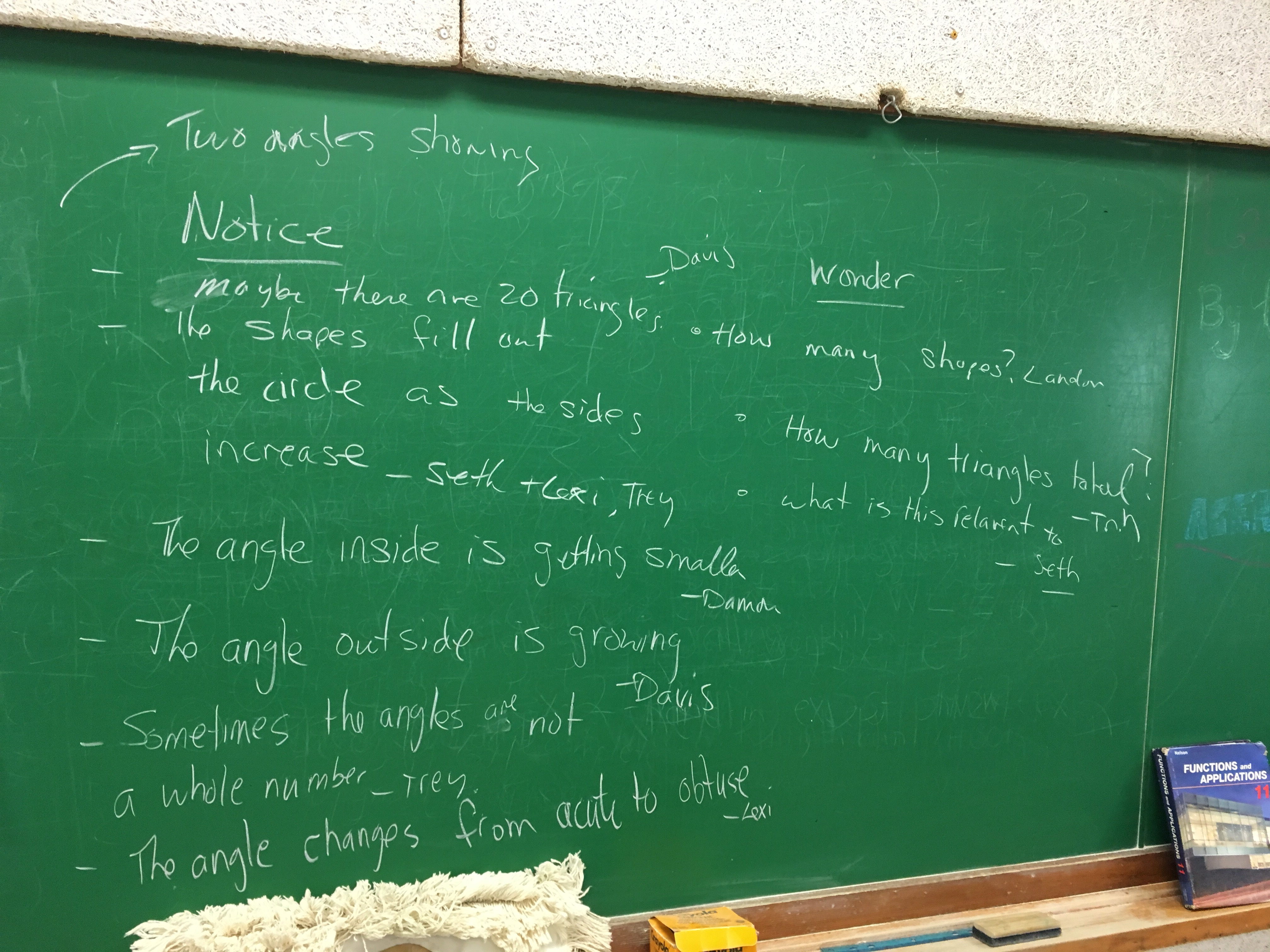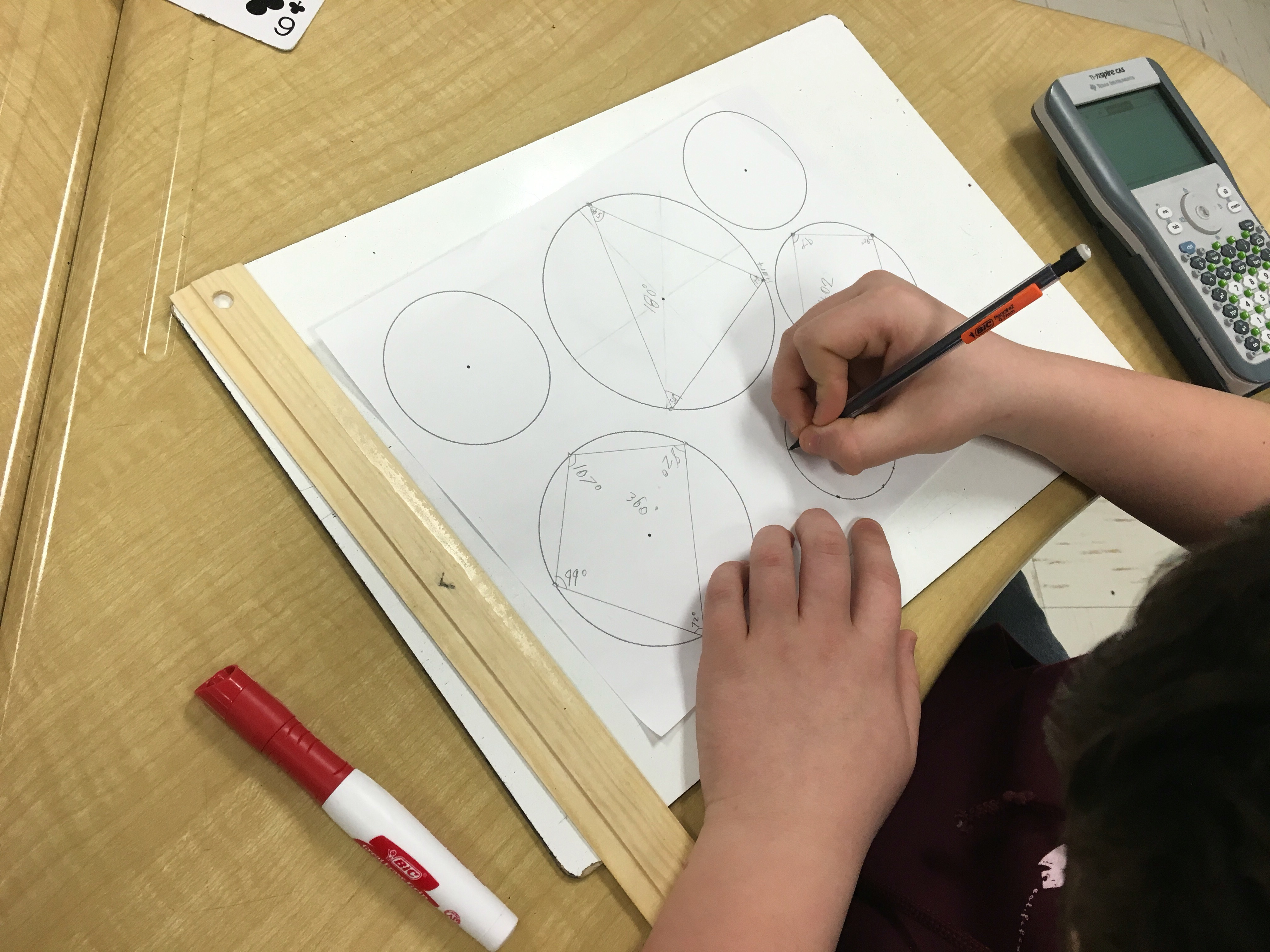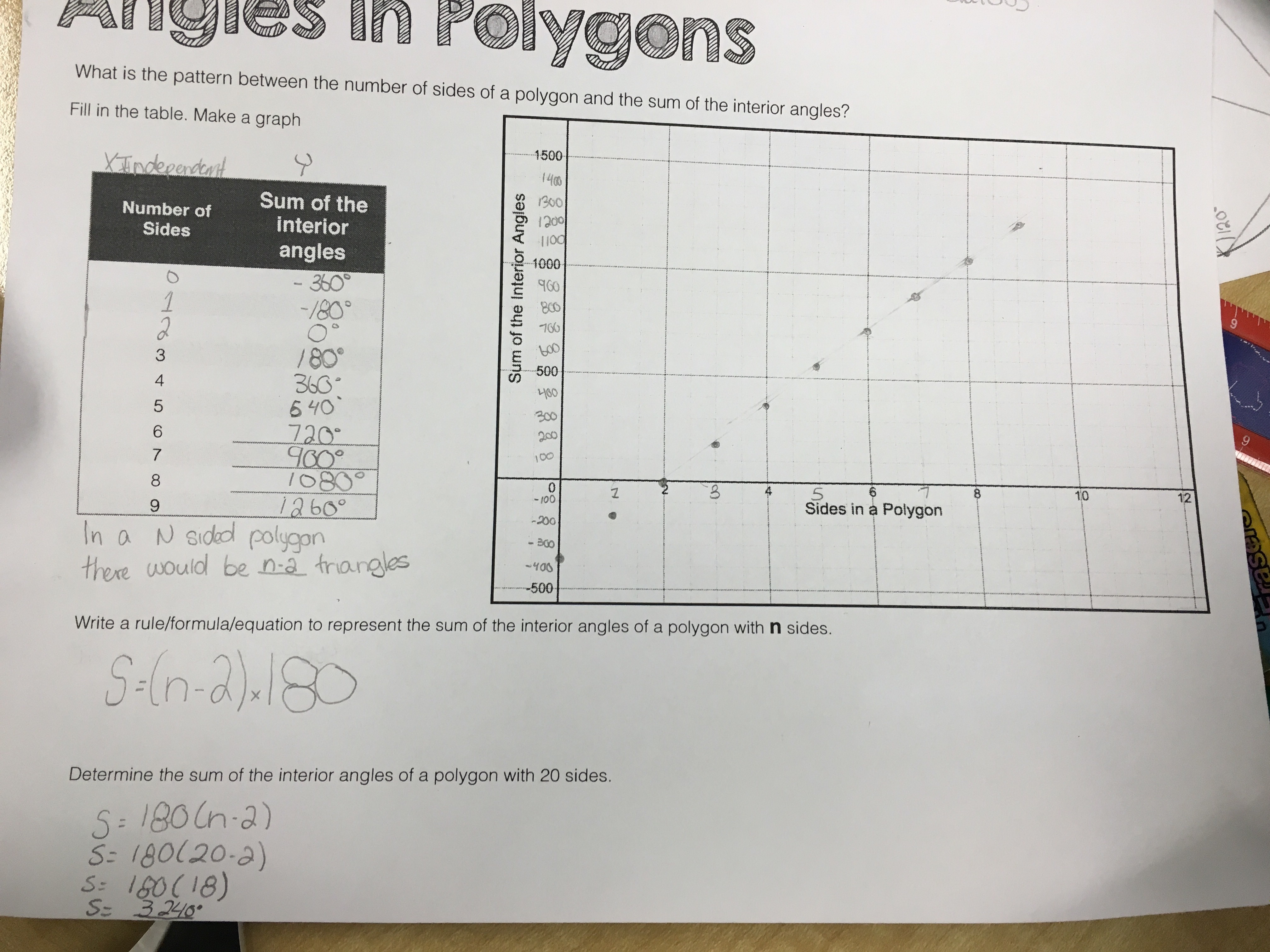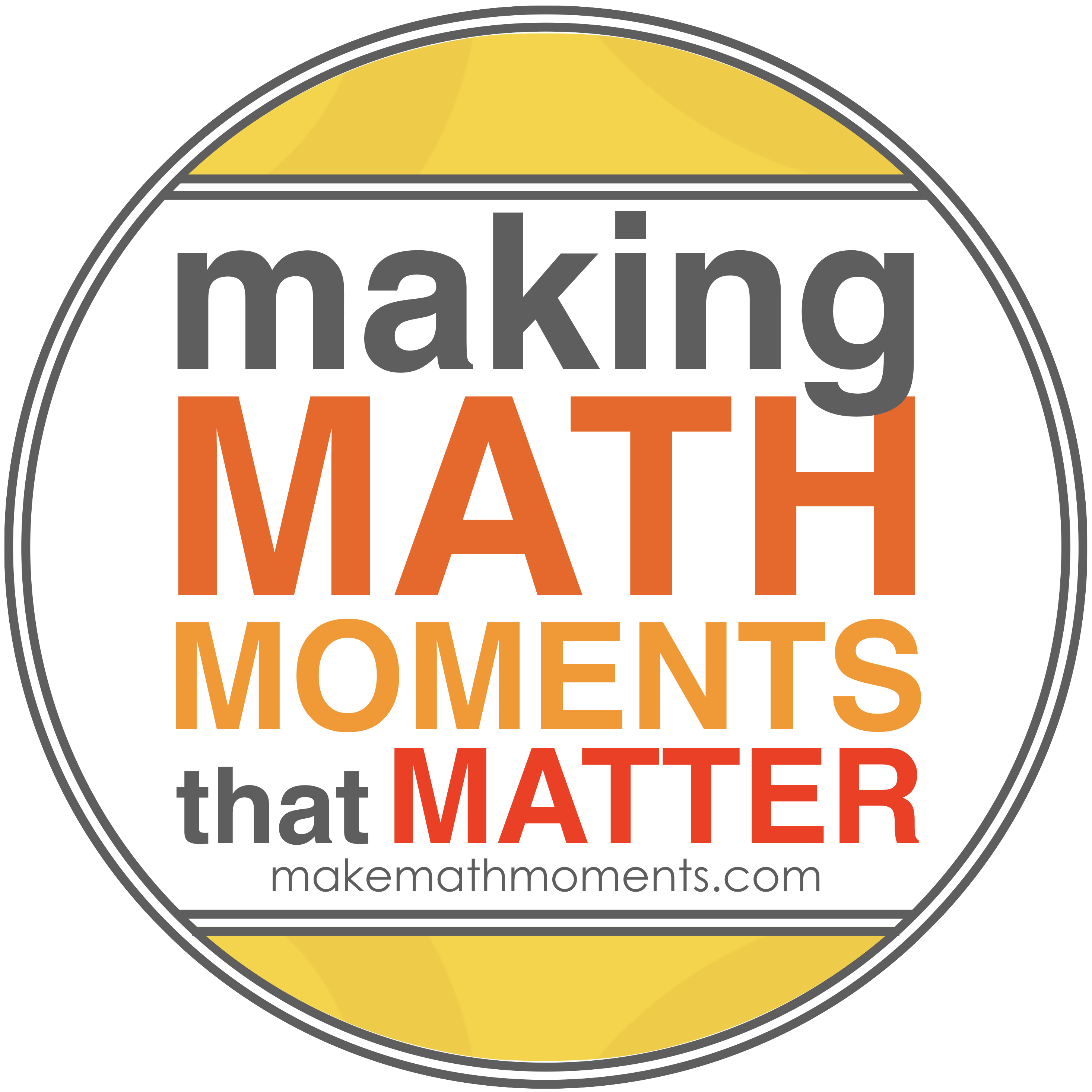# From Circles to PolygonsMy previous attempts at teaching the sum of interior angles in a polygon with grade 9 applied students went like this:

1. Day 1: Teach the sum of interior angles in a triangle. +practice problems
2. Day 2: Teach the sum of interior angles in a quadrilateral. +practice problems
3. Day 3: Use the pattern of triangles in a polygon to determine the formula for the sum of interior angles in a polygon. +practice problems.

I taught those lessons as if each is not related to the next!

Also, those lessons above had me doing most of the talking. I convinced myself that I was doing a discovery lesson with them…but I was really just showing/demonstrating to them that the angles added up to 180 or 360, etc

I wanted more doing!

Here was our progression this time around,

### Day 1: Circles to Angles

I showed this gif animation (not what I really wanted but I thought it would do the job).Then independently I had them answer: What do you notice? and, What do you wonder? After 2 minutes they shared with their partner. After 1 more minute they shared with the class.We used their noticing and wondering a to have a discussion on polygons, regular polygons, and acute vs. obtuse angles.

I posed the question:

“What would the interior angle be in a regular polygon if it had 20 sides, 30, 50?

I emphasized that looking for patterns can help us generalize. We set out on a path to find out what the sum of the interior angles for 3,4,5,6,7,8 sided figures…maybe we can see a pattern.
I handed out a page with 8 circles of varying sizes.I asked them to close their eyes and randomly plop their finger down on a circle.

I asked them to place a point randomly on the circumference of the circle. And then to add two more anywhere on the circle.

We connected the points up…at which time we talked about convex polygons.I handed out protractors. Measure the angles and write the sum inside the shape.

“How likely is it that any of drew the same triangle?”
Class: “None!”
I drew a giant triangle on the board and asked them to go up and put their sum inside the triangle.
Next they drew quadrilaterals, pentagons, hexagons, etc in the circles, measured angles, and calculated sums.By the end of day 1 our board looked like this.

###Day 2: Looking for Patterns

We looked at the sums placed in the shapes on the board for the triangle. There were lots close to 180 but not a lot exactly 180.

They seemed to remember that 180 was important and thought that all 3 angles should add to it.

I had them cut their triangle out. Cut each corner off…and try to arrange the pieces to make a half circle.

Boom! 180 degrees. On to the quadrilateral.

They guessed that the sum of the interior angles for a quadrilateral was 360 but weren’t sure why.
I asked them to pick a point on the quadrilateral and draw 1 line to make two triangles.

We agreed that each triangle’s interior angles add to 180 and there were 2 of them…then 2×180 is 360.
I handed out a sheet to keep track of the is potential pattern.We added those shapes to the sheet and plotted the points on the graph.

We divided the pentagon and remaining shapes into triangles and filled out the tableWe saw a definite pattern. A linear pattern.

As a class:

We found the first differences. We wrote a rule based on the number of triangles and related it to the number of sides in the polygon.

We then found the equation using the rate of change and y-intercept from the table.
We finally used the equation to determine the sum of interior angles for a 20 sided polygon…then found what the angles would be in a regular polygon.

To assess the students they completed practice problems on finding missing angles for varying shapes for the last 20 minutes while I circulated.

This course is all about patterns….use the patterns and prior knowledge to our advantage!

## 4 thoughts on “From Circles to Polygons”

1.Looks good! I’ve already taught these topics this year, but not this way. What a fun thing to before finals or as review/spiraling. Thanks!

1.Jon Orr

Thanks David. I’ve used sketches mostly in the past too. I like this time how we crowd sourced most of this lesson!

2.Gayoung

I like this activity! It is a nice way for students to find what the sum of the interior angle is without having us tell them. It also gives an opportunity for students to generalize the equation. I like how you had them plot points on graph to show that each sum are linearly related. If I have time, I would definiately try to implement this activity in my lesson! Thanks for sharing!## CONTACT ME